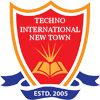# Antenna and Microwave Lab

EM THEORY AND TRANSMISSION LINES LABORATORY

Code: EC491

List of Experiments:

[At least THREE experiments from Module I and FOUR experiments from Module II]

Module I:

1. Plotting of Standing Wave Pattern along a transmission line when the line is open-circuited, short-circuited and terminated by a resistive load at the load end.

2. Input Impedance of a terminated coaxial line using shift in minima technique.

3. Study of Smith chart on Matlab platform.

4. Simulation study of Smith chart - Single and double stub matching.

Module II:

5. Radiation Pattern of dipole antenna.

6. Radiation Pattern of a folded-dipole antenna.

7. Radiation pattern of a 3-element Yagi-Uda Antenna.

8. Beam width, gain and radiation pattern of a 3-element, 5-element and 7-element. Yagi-Uda antenna - Comparative study.

9. Radiation pattern, Gain, Directivity of a Pyramidal Horn Antenna.

10. Study of Spectrum Analyzer.

RF & MICROWAVE ENGG LABORATORY

Code: EC793A

List of Experiments:

1. Determination of phase and group velocities in a waveguide carrying TE10 Wave from Dispersion diagram [ω−β Plot].

2. Measurement of unknown impedance using shift in minima technique using a waveguide test bench/ Measurement of the susceptance of an inductive and or a capacitive window using shift in minima technique using a waveguide test bench.

3. Study of the characteristics of a Reflex Klystron oscillator.

4. Study of Gunn-oscillator Characteristics using X-band waveguide test bench.

5. Measurement of coupling factor, Directivity, Insertion loss and Isolation of a Directional coupler using X-band waveguide test bench set up.

6. Scattering matrix of a magic tee / E-plane tee / H-plane tee using waveguide test bench at X-band.

7. Experimental/Simulation Study of filter (LPF, HPF, BPF) response.

8. Measuring of dielectric constant of a material using waveguide test bench at X-band.